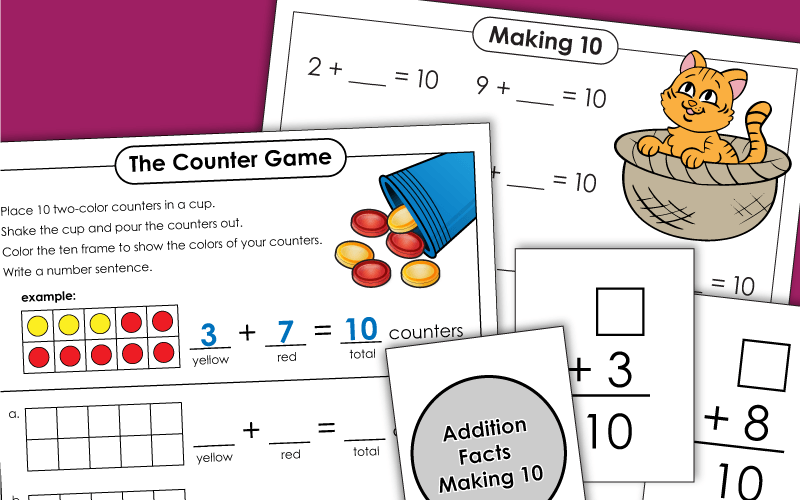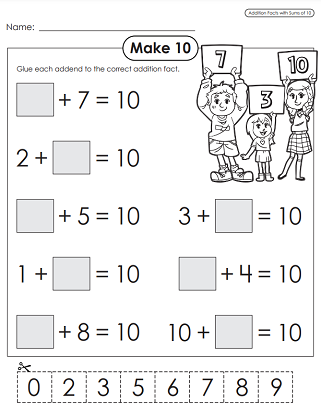On these printable worksheets, students will learn and practice "making 10." These are basic addition problems with sums of that equal 10.## Craft

Follow the instructions to color, cut, and glue this rainbow. Number flaps at the bottom help reinforce the understanding of addends used to make sums of 10.

## Game

Put 10 two-color counters in a cup and shake. Pour the counters on the table. Show the addition equation that has 10 as a sum.

## Worksheets

Count the red and white counters. Find out how many of each are added together to make 10.
With this worksheet, students can practice making 10 by cutting and gluing addends into the appropriate blank space in the math problem.
Color in a rainbow and use the numbers on it to solve the missing addend problems.
Tell how many more counters are needed to make 10 on each frame.
Write two addition and two subtraction facts for each ten frame shown.
Here's a blank ten frame template you can print for your students.

## Flash Cards

Students find the missing addend for each flash card.

Write the missing addends in the blank spaces to make sums of 10. Includes illustrations of cats that students can color in.
Students fill in the missing addends to make sums of 10. Features illustrations of food that can be colored in.
Ten Frame Worksheets

## Sample Worksheet ImagesMy Account
Site Information